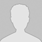>> 更多

### MySQL统计函数记录 按月、按季度、按日、时间段统计

select sum(mymoney) as totalmoney, count(*) as sheets from mytable group by date_format(col, '%Y');

select sum(mymoney) as totalmoney, count(*) as sheets from mytable group by date_format(col, '%Y-%m');

select sum(mymoney) as totalmoney,count(*) as sheets from mytable group by concat(date_format(col, '%Y'),FLOOR((date_format(col, '%m')+2)/3));
select sum(mymoney) as totalmoney,count(*) as sheets from mytable group by concat(date_format(col, '%Y'),FLOOR((date_format(col, '%m')+2)/3));

select sum(mymoney) as totalmoney,count(*) as sheets from mytable group by date_format(col, '%Y-%m-%d %H ');

SELECT * FROM mytable WHERE year(FROM_UNIXTIME(my_time)) = year(curdate())

SELECT id, quarter(FROM_UNIXTIME(my_time)) FROM mytable;

SELECT * FROM mytable WHERE quarter(FROM_UNIXTIME(my_time)) = quarter(curdate());

select * from mytable where month(my_time1) = month(curdate()) and year(my_time2) = year(curdate())

select * from mytable where month(my_time1) = month(curdate()) and week(my_time2) = week(curdate())• 全部评论（0
还没有评论，快来抢沙发吧！